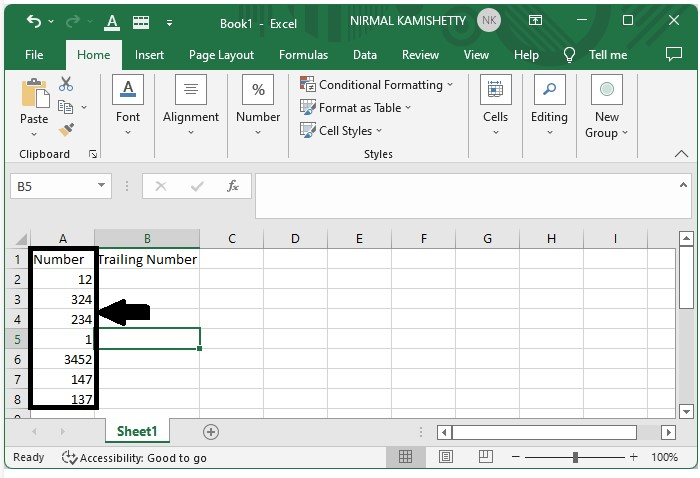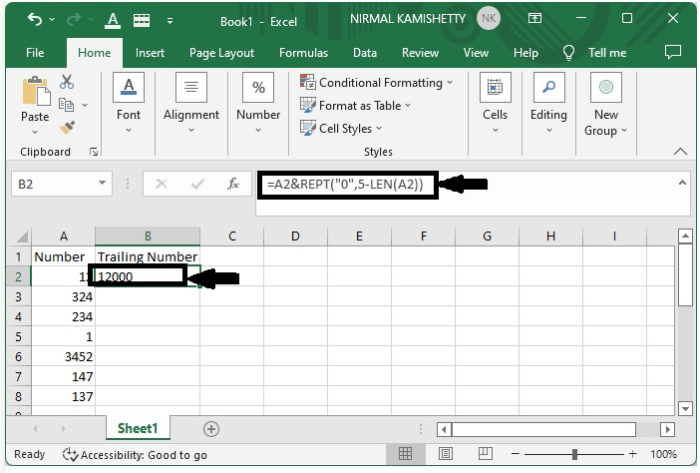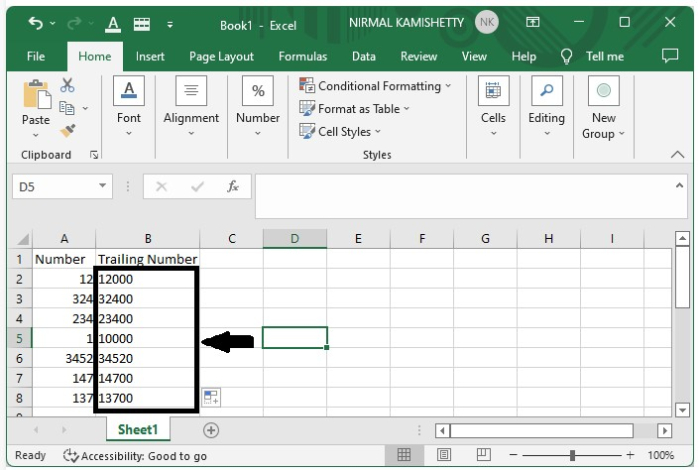# How to Add Trailing Zeros to a Column of Numbers in Excel?

Trailing zeroes are the zeroes that can be added after the number, which do not affect the value of the number. Some of the cases where we use trailing zeroes are when we calculate the mathematical values. In these cases, we can add the trailing zeroes after the dot in floating point numbers, which will not change the value of the number.

The primary purpose of using trailing zeroes is to ensure that all of the numbers in the data have the same number of digits. not zeros; we can add any number after the number using this same process. In this tutorial, let's see a simple process to know how we can add trailing zeros to a column of numbers in Excel.

## Adding Trailing Zeros to a Column of Numbers in Excel

Here, we will first use the REPT formula to get our first result, then use the auto-fill handle to fill in all the results. Let us see a simple process to add the trailing zeroes to the column of numbers.

Step 1

Consider the following Excel sheet with data similar to the image below.Step 2

To add trailing zeros, click on an empty cell and enter the formula as

=A2&REPT("0",5-LEN(A2)) in the formula box and click enter to get the first result.Step 3

Drag down from the right corner of the result to get all the remaking results and to produce our final result, as shown in the below image.Tips to Understand the Formula −

We have used the following formula −

=A2&REPT("0",5-LEN(A2))

Here,

• A2 = Current number's address

• REPT means to repeat the number

• 0 = to include the zero

• 5-LEN(A2) = until A2 reaches the length of 5

## Conclusion

In this tutorial, we used a simple example to demonstrate how we can add trailing zeros to columns in Excel to highlight particular sets of data.

Updated on: 09-Jan-2023

7K+ Views